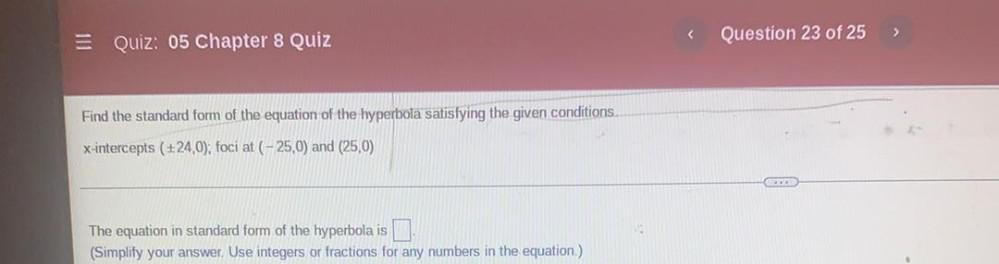Question:

# Quiz: 05 Chapter 8 Quiz Question 23 of 25 Find the standard form of the equation of the hyperbola satisfying the given conditionQuiz: 05 Chapter 8 Quiz Question 23 of 25 Find the standard form of the equation of the hyperbola satisfying the given conditions. x-intercepts (+24,0); foci at (-25,0) and (25,0) The equation in standard form of the hyperbola is (Simplify your answer. Use integers or fractions for any numbers in the equation.)